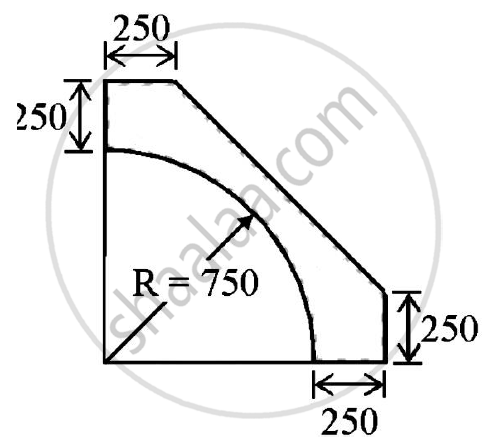# Find the Centroid of the Shaded Portion of the Plate Shown in the Figure. - Engineering Mechanics

Find the centroid of the shaded portion of the plate shown in the figure.#### Solution

Y = X is the axis of symmetry
The centroid would lie on this line

 Sr.no. PART AREA(in mm2) X co-ordinate(mm) Ax(mm3) 1. RECTANGLE =1000 X 1000=1000000 1000/2 = 500 500000000 2. TRIANGLE (to be removed) 1/2 X 750 X 750= -281250 1000 –750/2= 750 -210937500 3. QUARTER CIRCLE (To be removed) (pir^2)/4= 441786.4669 (4 x 7509)/(3pi)= 3141.5926 -140625000 TOTAL 276963.4669 148437500

overlineX =(Σ Ax)/(Σ A)=(148437500)/(276963.5331)=535.946 mm
𝑦̅ = 𝑋̅ = 535.946 mm
CENTROID IS AT (535.946,535.946)mm

Concept: Centroid for Plane Laminas
Is there an error in this question or solution?

Share